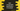# Dart comparable example for comparing objects## How to use Comparable for comparing objects in Dart :

Comparing two custom object can be done using Comparable interface. If you are familiar with Java, it works in a similar manner. In this tutorial, I will show you two examples of how to use it in Dart. Suppose, we have a list of students and we want to compare two students based on their marks. Our program will compare any two of the students and print out the result (which one got the highest mark) :

``````//1
class Student implements Comparable {
final String name;
final int marks;

//2
const Student(this.name,this.marks);

//3
@override
int compareTo(Student anotherStudent) => marks - anotherStudent.marks;
}

main(List args) {
//4
var alex = const Student("Alex",80);
var albert = const Student("Albert",95);

//5
if(alex.compareTo(albert)){
print("Alex got more marks than Albert");
}else{
print("Albert got more marks than Alex");
}
}``````

### Explanation :

The commented numbers in the above program denote the step number below :

1. Student class represents a student. It has two values: name to hold the name of the student and marks to hold the marks of a student. We are implementing the Comparable interface for this class.
2. This one is the constructor for the Student class. We need to pass the name and marks of a student to create a Student object.
3. compareTo method is required to override if we are implementing Comparable interface. It will compare one object’s property with the current object. Here, we are checking current student mark - another student mark. If the mark of the current student is more than another student, it will return true. Else, it will return false.
4. To test the implementation, we have created two Student objects alex and albert first.
5. Then, we have compared both using the compareTo method. If the marks of alex are more than albert, it will print the line inside if block. Else, it will print the second print statement.

### Output :

``Albert got more marks than Alex``

### Comparing more than one values :

We can compare more than one values inside the compareTo method. Instead of only comparing, we can also write any logic inside this method. Example :

``````class Student implements Comparable {
final String name;
final int marksA;
final int marksB;
final int marksC;

const Student(this.name, this.marksA, this.marksB, this.marksC);

@override
int compareTo(Student anotherStudent) {
if (marksC < anotherStudent.marksC) { return false; } if (marksA + marksB > anotherStudent.marksA + anotherStudent.marksB) {
return true;
}
return false;
}
}

main(List args) {
var alex = const Student("Alex", 20, 30, 10);
var albert = const Student("Albert", 25, 30, 10);

if (alex.compareTo(albert)) {
print("Alex got more marks than Albert");
} else {
print("Albert got more marks than Alex");
}
}``````

It will print :

``Albert got more marks than Alex``

### Conclusion :

Comparable comes in handy while dealing with custom objects. You can implement any complex logic to compare two objects however you want. Try to run these examples and drop a comment below if you have any queries.